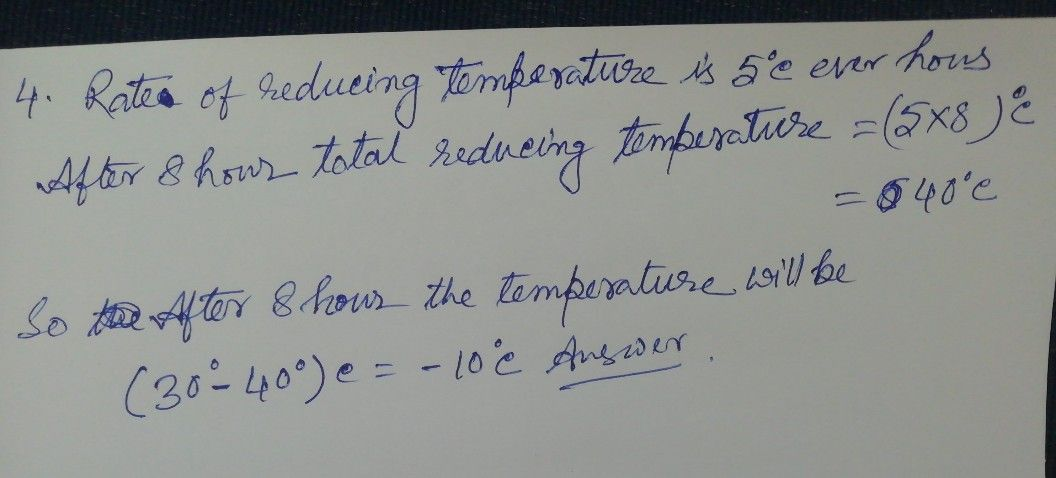Symbol
Problem$x$ withdrawal. $4$ For an experiment, the temperature of a liquid is to be ri to noieiv lowered from $30^{°}C$ at the rate of $5^{°}C$ every hour. What will be the temperature of the liquid $8$ hours after the process begins? $5A$ A $0$ company is producing two products $AA$ and $AI$ The o om molkog profit of
7th-9th grade
Other
SolutionQanda teacher - himadri007any doubt please ask me